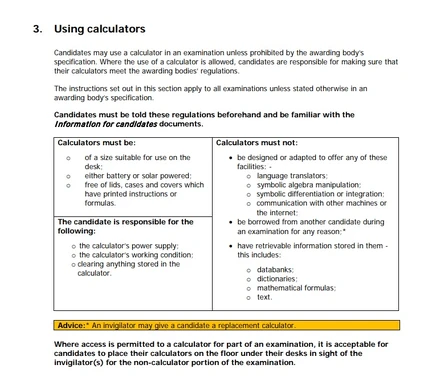120 Pages

## Which calculators are allowed in UK exams?EditJCQ Instructions for Conducting Examinations 2017-2018, Calculator regulations - p13

Your choice of calculator depends on the level of the exam.

This page is aimed at people taking maths exams. If you are not taking maths yet, but are taking science exams, then you may be able to manage with a basic calculator (a non-scientific calculator).

For GCSE and IGCSE Maths Higher level, you will need a scientific calculator which can do trigonometric functions, square roots etc, whilst for A-levels you will need one which can do certain probability functions.  If you expect to do A-level maths then you can buy a more advanced calculator straight away, but bear in mind that the more complex calculators require more investment of time in learning how to use them properly.

See the image for UK exam rules on calculators, taken from p13 of the JCQ Instructions for Conducting Examinations 2017-18 . JCQ is the Joint Council for Qualifications - the body which sets the rules for running GCSEs and A-levels in the UK.

## GCSE CalculatorsEdit

Models commonly used for GCSE which can be bought on the high street include:

• Casio FX-83 GT Plus
• Casio FX-85 GT Plus (same as above but with solar power for backup)

No wifi-enabled calculators.

No data storage facility eg dictionaries, text storage, mathematical formulae.

If the calculator has a case then this must be removed (in case notes are written on it).

AQA's advice on GCSE maths calculators - here's an extract:

For GCSE Mathematics exams, calculators should have the following as a minimum requirement:

• four rules and square
• square root
• reciprocal and power function
• brackets
• a memory facility
• appropriate exponential, trigonometric and statistical functions.

For the purposes of this specification, a ‘calculator’ is any electronic or mechanical device which may be used for the performance of mathematical computations. However, only those permissible in the guidance in the Instructions for conducting examinations are allowed in GCSE mathematics examinations.

## A-level Maths CalculatorsEdit

For A-level maths you can use a more advanced calculator for UK A-levels. This includes some graphical calculators, which can save you time if you learn to use them properly. However, some models are banned!

MEI article on A-level maths calculators is a highly recommended page including all the advice you need, including tutorials for common models so that you can get the most out of the calculators. They say:

For the 2017 A levels students will require a calculator that can calculate Binomial and Normal probabilities directly from values. The minimum standard for this is an advanced scientific calculator, such as the Casio 991EX ClassWiz or the TI-30X Pro; however, graphical calculators have this facility along with the additional advantage of being able to plot the graphs of functions. Graphing tools should be permeating the study of A level and integrating graphical calculators into the teaching and learning is a very effective way of achieving this.

The Truth about Calculators for Maths A-Levels, from OCR 2017 - great article clarifying lots of points, and explaining where you can economise if you can't afford a fancy calculator.

OCR guidance and FAQs on calculators for A-level maths is also well worth a read. Here are some extracts:

### What about graphical calculators?Edit

Graphical calculators are permitted in exams (but not Core 1), as long as they meet the JCQ regulations. In the MEI specification there are optional topics in Further Pure 2 (Investigation of curves) and Further Pure 3 (Markov chains) for which a graphical calculator with the right features is essential. For other units a graphical calculator is not essential, but it may be helpful if a candidate has used one throughout the course.

### What about programmable calculators?Edit

Programmable calculators are permitted in exams, and candidates may use the programming facilities during the exam. However there must not be any programs stored in the calculator at the beginning of the exam; this is the bit in the JCQ regulations about ‘retrievable information’. It is good practice for the centre to check this at the beginning of the exam; for example invigilators could ask candidates to show them that the memory assigned to programs is zero.

### Suggested calculators for A-levelEdit

These calculators have been recommended for A-level maths as they includes Binomial and normal distribution functions. All are commonly used in A-level maths exams so should not be controversial:

Texas Instruments TI-30X Pro - around £20 here.

Casio FX-991EX ClassWiz Scientific Calculator - under £30, available on high street.

Casio FX-9860GII Advanced Graphic Calculator - about £90

Maths teachers' recommendations from TES

Edexcel guidance on A-level maths calculators from 2016 onwards

Note that CIE (Cambridge International Examinations) have slightly different regulations and ban all graphical calculators for their A-levels. However, this is under review so check as exceptions may be made for a specific syllabus.

Read more on Making entries and sitting exams.